# Proton 3: 2nd Degree, Polar Star (P3-20P)The Proton Shell of 3 is built from nine hadron strings. Three of the strings are "structural," meaning they form the core structure, or rather, the shape of the particle. Other solution sets for the P3 shell can be devised, but of the solution sets I have found thus far, all are built on the same pyramidal core of three large structural loops. Shown here is what I believe to be the "ground state" of P3. I have stacked this shell with N4-22P to form a model of the Lithium atom. (See below for a couple of interesting "third degree" solutions for P3.)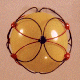## Stereograms of P3-20P Shell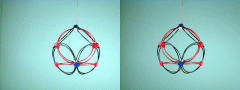Required Snap Points: 30 Available Snap Points: 30 Pinned Vector Bosons: 0 L1 = 20.3036972" (Structural) L2 = 15.3481514" (Filler) Model Diameter: 7.462673154" Loop Ratio (1:2): 1.322875744 Loop Equation: 3(L1) + 6(L2) = 153" Snap Point Equation: 3(4) + 6(3) = 30 R.S.P.

# Proton 3: 3rd Degree, Polar Star (P3-30PS)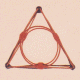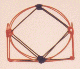A third degree solution set for P3... Note the three Loop values, and in particular, the unusual sinusoidal string. I have my doubts as to whether this particular model represents a valid solution set, but it does not violate any rule for nuclear geometry that I have thus far devised. In spite of its unusual shape and unique structure, this model has been extremely valuable to me. It was this particular model that inspired me to mensurate the loop values of the nucleus by their common chords, greatly simplifying the math required to describe the physical structure of the models. This, coupled with the model for P5 (boron), in turn led to the discovery of what I call the "polar star" solutions... You'll see more about them as you examine higher nuclear shells.

## Stereograms of P3-30PS Shell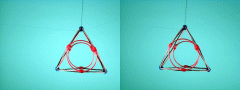Required Snap Points: 30 Available Snap Points: 30 Pinned Vector Bosons: 0 L1 = 22.31736249" (Structural) L2 = 12.88492923" (Polar) L3 = 60.27805408" (Box Sinusoidal) Model Diameter: 7.103837113" Loop Ratios: (1:2): 1.732051615 (1:3): 0.370240261 (2:3): 0.213758215 Loop Equation: 3(L1) + 2(L2) + 1(L3) = 153" Snap Point Equation: 3(4) + 2(3) + 1(12)= 30 R.S.P.

# Proton 3: 3rd Degree, Polar Star (P3-30PA)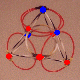A third degree solution set for P3... this one is probably more likely to arise than the third degree solution given above. The smaller polar circlets replace two of the filler circlets in the first P3 shell given on this page. This modification of the geometry results in a different quark distribution for this P3 variant. Another similar solution (below) having a single small polar circlet yields yet another quark distribution. Such is the first real clue to the fluid nature of nuclear geometry. Which shell actually gets used in a typical lithium atom probably depends largely on the neutron shell which is combined with it. I presume that the quark distributions between shells will experience strong charge coupling, promoting more complex shell structures to stability due to charge incompatibilities with simpler shell solutions. As you can see, when working with individual proton or neutron shells, nuclear geometry is rather simple and elegant, but when you start stacking the shells, things start getting complicated real fast. This aspect of the work is best done by a computer analysis of shell solution sets. Unfortunately, no software exists that can do the analysis, at least not yet.

 Required Snap Points: 30 Available Snap Points: 30 Pinned Vector Bosons: 0 L1 = 21.31389556" (Structural) L2 = 16.11178953" (Filler) L3 = 12.3055776" (Polar) Model Diameter: 7.833974012" Loop Ratios: (1:2): 1.322875744 (1:3): 1.732051615 (2:3): 1.309307864 Loop Equation: 3(L1) + 4(L2) + 2(L3) = 153" Snap Point Equation: 3(4) + 4(3) + 2(3)= 30 R.S.P.

# Proton 3: 3rd Degree, Polar Star, 1-Polar (P3-30PB)

No Picture Available

 Another third degree solution set for P3... this one is almost identical to the preceding model except that one of the polar circlets has been removed and replaced with a filler circlet, resulting in a different quark distribution.

 Required Snap Points: 30 Available Snap Points: 30 Pinned Vector Bosons: 0 L1 = 20.79653593" (Structural) L2 = 15.72070244" (Filler) L3 = 12.00688002" (Polar) Model Diameter: 7.643817225" Loop Ratios: (1:2): 1.322875744 (1:3): 1.732051615 (2:3): 1.309307864 Loop Equation: 3(L1) + 5(L2) + 1(L3) = 153" Snap Point Equation: 3(4) + 5(3) + 1(3)= 30 R.S.P.

Copyright 1997 by Arnold J. Barzydlo
Go Back